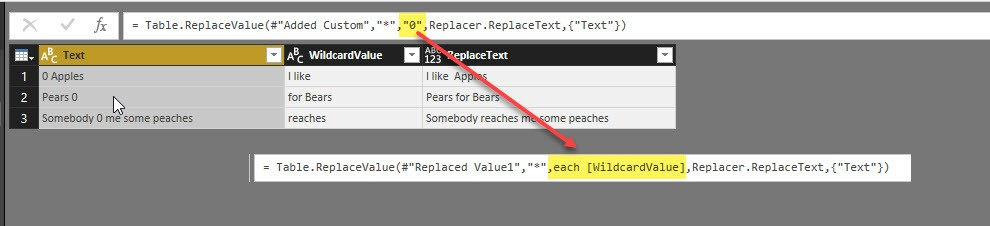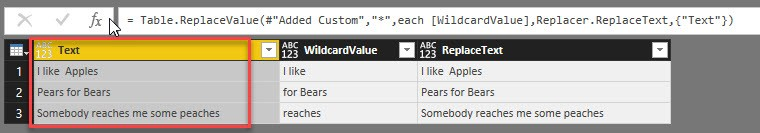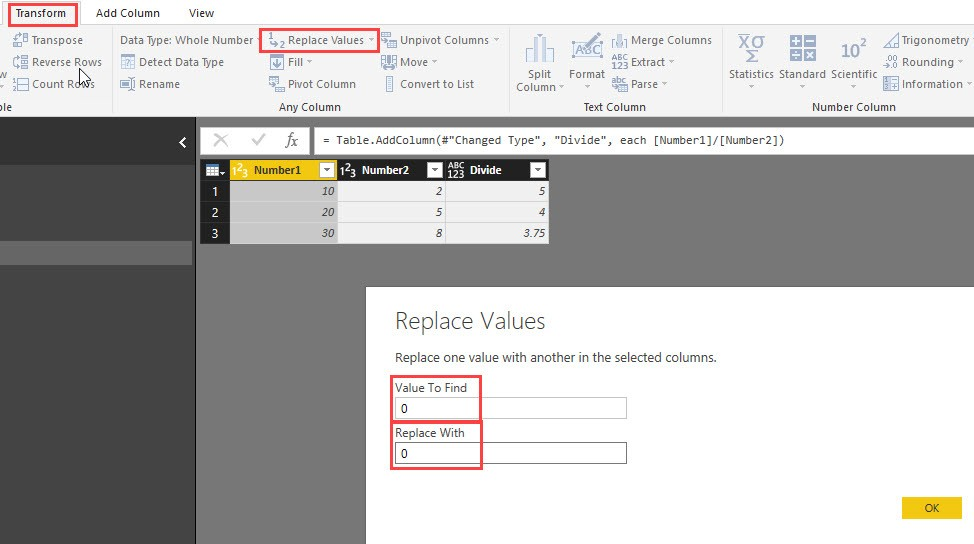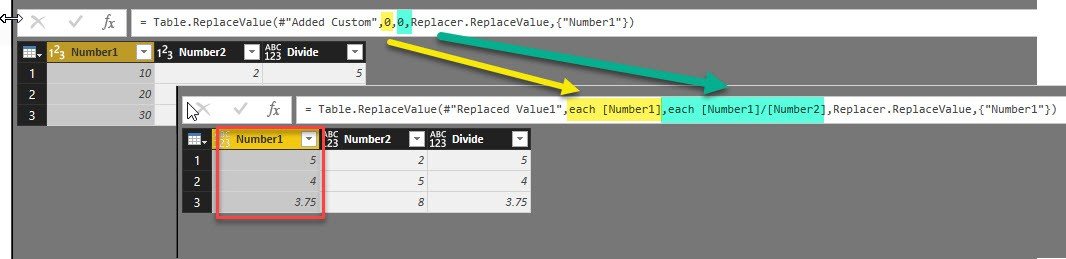# Table.TransformColumns – alternative in PowerBI and PowerQuery in Excel

This article shows a trick for a little problem that annoyed me for quite some time: How to get Table.TransformColumns transforming the values of a column with a reference to a value (from the same row) of another column?

### 1 Replace text by a value from another column

So instead of adding a new column where the “*” is replaced by the value from column “WhildcardValue”, I just want to perform the replacement-operation in the original “Text”-column, so that I don’t have to rename and delete the other columns later:So far, I always ended up fighting with Table.TransformColumns-function and got quite frustrated because I couldn’t find a way to reference the (row-) value of the other column. As it turns out, I was fighting the wrong target here, because Table.ReplaceValue is actually the saviour for this challenge:

##### 1.1 Check column “Text” -> Transform -> Replace ValuesSo in (4) we just filled in a dummy-value, which we’re going to replace with a reference to the desired column: “each [WildcardValue]” like this:

##### 1.2 Edit the generated function code in the formula editor:`Code: = Table.ReplaceValue(#"Replaced Value1", "*", each [WildcardValue], Replacer.ReplaceText, {"Text"})`That’s cool.

But we can top it:

### 2 Divide [Number1] by [Number2]:##### 2.1 Again: Check column “Number1” -> Transform -> Replace Values, but fill in dummies for both:##### 2.2 Edit 2 fields in the formula editor:Watch the 2nd replacement: We just need one “each” at the beginning of the expression, then the references to the columns just need the square brackets like

`Code: = Table.ReplaceValue(#"Replaced Value1", each [Number1], each [Number1]/[Number2], Replacer.ReplaceValue, {"Number1"})`

One thing to mention: This command is sensitive to the type of the column where it is called from. So in the first example, where we called it from a text-column, the 4th argument of the function has automatically been set to “Replacer.ReplaceText”. But in the 2nd example it is call called from a number-column and there it has been set to “Replacer.ReplaceValue”. This has served us well here, but can lead to unexpected errors in some other cases: When you want to replace the values of a text-column with a value from a number-column, you have to wrap the reference to the number-column into a “Text.From(…)”, to transform the number-value into a text-string.

Performance warning: Although you code looks cleaner using this method, it will most likely be slower than manually adding a column does the desired transformations, delete the old column and rename the newly created column.

Edit: … and here we go again: This trick was not new, one just need to search before posting 😉 : https://bondarenkoivan.wordpress.com/2016/12/12/transform-column-using-custom-function-in-power-query-part-2/ (in Miguel Escobars comment below)

Enjoy & stay queryious 😉

### Comments (19) Write a comment

1.With the Divide option – would you normally use

= Table.AddColumn(PreviousStep, “New Column Name”, each [Item 1] / [Item 2], type number) ?

•Thanks Wyn!
Personally, I’d end up without the type-definition at the end, because I’m always using the UI for this step and forget to transform there.
But the definition for sure makes sense, as you don’t have to transform column types later.
Actually, I whish that this type would be defined automatically for numbers, once one has done mathematical calculations.

2.Thanks. I had a same problem many times, and my solution was to use AddColumns, although I knew about Table.ReplaceValue function. I was blinded with the old habit. There is also a function Table.ReplaceMatchingRows which could come handy if you wish to fix several columns at once.

•Thank you & yes: We still miss the transformation for multiple columns.
Table.TableReplaceMatchingRows seems to require a match-definition of all columns, or am I mistaken here?

3. Pingback: #Excel Super Links #107 – shared by David Hager | Excel For You

4.A problem with Table.ReplaceValue is after the transformation all columns change data type to any – I wonder what is the logic behind that

5.Imke, did you ever find a way to reference the current row using TransformColumns? Thnx. Wouter

•Hi Wouter,
no, I didn’t. Actually: I don’t expect this to be possible, so I have stopped searching.

6.Greetings!

Code: = Table.ReplaceValue(#”Replaced Value1″, “*”, each [WildcardValue], Replacer.ReplaceText, {“Text”})

how can “each [WildcardValue]” be used in order to reference a column to another query?

•You need a row/record-context here. So I cannot see how referencing a whole column in a different query can work in this case.

7.I tried to follow and couldn’t get it and I don’t know where I am failing — I am trying to replace column A value 31 to 19 if column B is biller name “VTNS”. Also need to change column a value 10 to 1 if column B biller name is “VTNS” else whatever value is in A needs to remain. I have tried multiple forums and told to try an add column function but am getting syntax errors and errors that the added column doesn’t exist.

Here’s what I’ve been working with. Honestly, I want to understand it and get it functioning. I was only able to get one else statement and couldn’t figure how to get the rest.

Table.ReplaceValue(#”New Column”, each [BILLING_PERIOD_CD], each if [Biller Name Lookup.Biller Name] = “VTNS” and [BILLING_PERIOD_CD] = “31” and then “19” else [BILLING_PERIOD_CD],Replacer.ReplaceValue,{” BILLING_PERIOD_CD “})

•Hi Elizabeth,
cannot think how this can be done with this method.
But no worries: Adding a column and write out the conditional expression will probably not slow down your query.

•I think you’re misunderstanding this process. it doesn’t add a new column, it keeps the column in place and changes the value.
Your function should look something like this where #”Previous Step” is the name of the the step prior to the replacement:

= Table.ReplaceValue(#”Previous Step”,each [BILLING_PERIOD_CD],each if [Biller Name Lookup.Biller Name] = “VTNS” and [BILLING_PERIOD_CD] = “31” then “19” else if [Biller Name Lookup.Biller Name] = “VTNS” and [BILLING_PERIOD_CD] = “10” then “1” else [BILLING_PERIOD_CD],Replacer.ReplaceValue,{“BILLING_PERIOD_CD”})

Provided that BILLING_PERIOD_CD is a Text type field. If It is not then remove the quotes from around the numbers.

Here’s a full M-Code where the column type is Text:

let
Source = Table.FromRows(Json.Document(Binary.Decompress(Binary.FromText(“i45WMjRQ0lEKC/ELVorViVYyNkTmgeX8QzxcgxCSjn5QgVgA”, BinaryEncoding.Base64), Compression.Deflate)), let _t = ((type nullable text) meta [Serialized.Text = true]) in type table [BILLING_PERIOD_CD = _t, #”Biller Name Lookup.Biller Name” = _t]),
#”Previous Step” = Table.TransformColumnTypes(Source,{{“BILLING_PERIOD_CD”, type text}, {“Biller Name Lookup.Biller Name”, type text}}),
#”Replaced Value” = Table.ReplaceValue(#”Previous Step”,each [BILLING_PERIOD_CD],each if [Biller Name Lookup.Biller Name] = “VTNS” and [BILLING_PERIOD_CD] = “31” then “19” else if [Biller Name Lookup.Biller Name] = “VTNS” and [BILLING_PERIOD_CD] = “10” then “1” else [BILLING_PERIOD_CD],Replacer.ReplaceValue,{“BILLING_PERIOD_CD”})
in
#”Replaced Value”

8.Hi Imke – I think I solve the Conditional Multiple Transformations

(By the way I enjoyed your interview on Power Query Magic) 🙂

What do you think of this?

let
Source = Table.FromRows(Json.Document(Binary.Decompress(Binary.FromText(“i45W8k5NLVDSUcomRMXqwNXm56TgJGNjAQ==”, BinaryEncoding.Base64), Compression.Deflate)), let _t = ((type nullable text) meta [Serialized.Text = true]) in type table [Column1 = _t, Column2 = _t, Column3 = _t, Column4 = _t, Column5 = _t, Column6 = _t]),
#”Changed Type” = Table.TransformColumnTypes(Source,{{“Column1”, type text}, {“Column2”, type text}, {“Column3”, type text}, {“Column4”, type text}, {“Column5”, type text}, {“Column6″, type text}}),
ConditionalTransform = Table.FromRecords(
Record.TransformFields(row,
List.Transform({“Column2″,”Column3”, “Column4”, “Column5″,”Column6”}, (name) =>
{name, each if row[Index] = 1 then “new” else _})
)
),
),
SelectiveTransform = Table.FromRecords(
(r) =>
Record.TransformFields(
r, {
{“Column2”, each if r[Index] = 0 then “new” else _},
{“Column3”, each if r[Index] = 1 then “new” else _}
}
)
),
)
in
SelectiveTransform

•Thanks, nice code!

9.